cancel
Showing results for
Search instead for
Did you mean: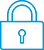It has been a while since anyone has replied. Simply ask a new question if you would like to start the discussion again.Level 1
11 6 0 0
Message 1 of 7
1,336
Flag Post

Solved!

# HP PRIME Line Integral (Biot-Savart Equation)HP Recommended
HP PRIME

Hello friends!

I`m trying to evaluate some integrals in this format:Some of my especifcs values are:

dl = [-a*sin(w)*dw , a*cos(w)*dw , 0] -> dw is the infinitesimal increment in w

R = [a*cos(w) , a*sin(w) , -z]

a=0

b=pi

I know how to operate the Cross Product of 2 generic vector, but, how can I solve this kind of integration when the infinitesimal vector is a operand of a cross product ?

Thank you for your time!

-Luiz Bernardi.

Tags (1)
6 REPLIES 6Level 9
3,551 3,490 180 541
Message 2 of 7
Flag PostHP Recommended

Hi!, @luiz_aug:

If you see, this link, you can learn and use in HP PRIME ... http://hyperphysics.phy-astr.gsu.edu/hbase/magnetic/Biosav.html

Kind Regards !.
Have a nice day !.
@Maké (Technical Advisor Premium - HP Program Top Contributor).
Provost in HP Spanish Public Forum ... https://h30467.www3.hp.com/Level 1
11 6 0 0
Message 3 of 7
Flag PostHP Recommended

Hi Maké!

I spent some time reading the link you suggested, and I got some positive results.

I choosed to work with the differential form instead of the integral form, as shown at the explanation. Here is what I did:

1- defined dB as the differential form of the Biot-Savart Law:

db:= (u*j*CROSS(diff([a*cos(w),a*sin(w),0]),w),[-a*cos(w),-a*sin(w),z]))/(4*pi*ABS([-a*cos(w),-a*sin(w),z])ˆ3)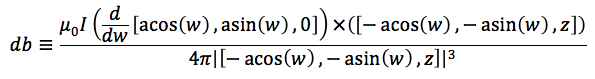2- Now that dB is set, and is a function of w, I found the correct results by doing:

int(db,w,0,pi)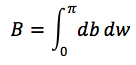But my main doubt is right here: Why cant I simply do something like this:I mean, I have already told the calculator that db is a diferential vector, why this operation gives me the answer pi as if I were doing the integration over a generic variable b ?

Another question that maybe helps to figure out how to do evaluate this kind of problem:

Is there some way to set the calculator to do integrals over a path, instead of doing it over a simple variable?

Tags (5)Level 6
305 297 32 76
Message 4 of 7
Flag PostHP Recommended

This is what I did.

So I replaced dl with v*dw.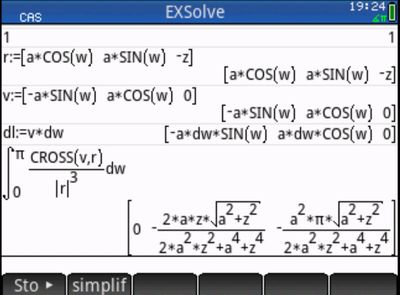Tags (1)Level 9
3,551 3,490 180 541
Message 5 of 7
Flag PostHP Recommended

Hi!, @luiz_aug:

See, the video and resolution with simplified calculus, from ... http://study.com/academy/lesson/the-biot-savart-law-derivation-examples.html

Try, with this equation's, from Wolfram Alpha ... http://www.wolframalpha.com/input/?i=Biot+Savart&rawformassumption=%7B"C",+"Biot+Savart"%7D+->+%7B"P...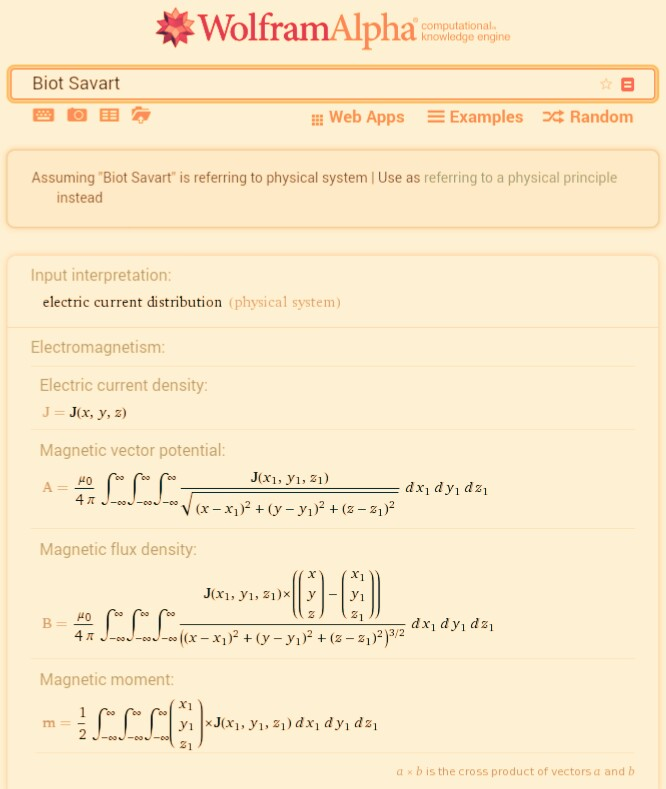Kind Regards !.
Have a nice day !.
@Maké (Technical Advisor Premium - HP Program Top Contributor).
Provost in HP Spanish Public Forum ... https://h30467.www3.hp.com/Level 1
11 6 0 0
Message 6 of 7
Flag PostHP Recommended

Hi Jan_D

First, thank you for your time answering me!

Let me see if I undestood your strategy:

You defined dl=v*dw, then by the scalar multiplication property of the vector product you assumed that
Cross(dl,r) = Cross(v*dw,r) = dw*Cross(v,r) ?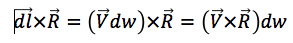Why did you defined dl if you dont use it at all ? Just to explicit that dl=v*dw ?

Thank you one more time!Level 6
305 297 32 76
Message 7 of 7
Flag PostHP Recommended

Yes, you understood me well.

It is correct that I did not have to define dl because I did not use it.

Like you said it only proved that dl=v*dw

† The opinions expressed above are the personal opinions of the authors, not of HP. By using this site, you accept the Terms of Use and Rules of Participation